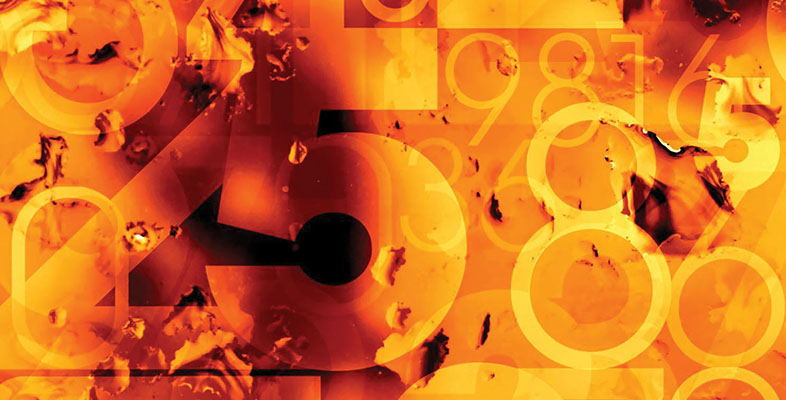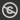Science, Maths & Technology
Free course

# Introduction to algebra## Course reviews

This free course is an introduction to algebra which builds on the idea of using letters to represent numbers. Section 1 looks at finding, simplifying and using formulas and includes useful tips on learning algebra. Section 2 introduces some of the terminology used in algebra and techniques such as evaluating and checking equivalence of expressions, identifying terms in an expression and collecting like terms. Section 3 looks at how to simplify terms and introduces index notation and multiplying powers. Section 4 looks at the use of brackets in algebra and section 5 covers solving, checking and using simple linear equations.

## Course learning outcomes

After studying this course, you should be able to:

• recognise technical terms and appreciate some of the uses of algebra
• collect like terms and simplify expressions term by term
• multiply out brackets
• simplify some formulas
• solve simple linear equations.

First Published: 27/09/2017

Updated: 18/04/2018

Skip Rate and Review